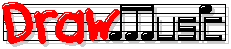Pop Music Theory

Lessons in Order

 1-7 (Intro & Pitch) +
 8-18 (Major Scale) +
 19-29 (Chord Progressions) -
 30-34 (Hook Chords) +
 35-41 (Written Notes) +
 42-50 (Song Chorus) +

Lessons by Topic

 Strategy +
 Pitches +
 Scales +
 Written Notes +
 Chords +
 Chord Progressions +
 Melody +
 Songwriting Steps +
 Science +
 Games & Tools +
 Song Examples: Crazy +
 Song Examples: Rolling Stone +

Detailed Contents

Get Future Lessons

Lesson 20: Roman Numeral Chords

This lesson teaches how to analyze chord progressions using Roman numeral chord symbols.

Before taking this lesson, you should know:

You learned how we can play a melody in any key in Lesson 19: Keys. And, we can show a melody in all keys at once by writing its notes as scale degrees (numbers) instead of actual pitch names.

This lesson shows how we can do the same thing with chords, by using Roman numeral chord symbols. You'll need to know Roman numerals, but just the numbers 1 through 6:

 Arabic numerals: 1 2 3 4 5 6 Roman numerals: I II III IV V VI

A Roman numeral chord symbol is like a regular chord symbol except that we use a Roman numeral instead of a pitch name to name the root of the chord. The Roman numeral is the chord's root, but it labels the root as its scale degree in the song's key.

A few "question and answer" examples are probably easier to understand than that definition:

Q: A song in the key of C uses a Dm ("D minor") chord. What is the chord's Roman symbol?

A: The pitch D (the chord's root) is scale degree 2 of a C major scale (the song's key). So the Roman numeral is II (for scale degree 2), and the complete answer is IIm ("two minor", which is just the original chord symbol, "Dm", replacing the "D" with "II").

Q: What is the IV chord in the key of E♭?

A: IV means the "four major" chord, so the chord's root will be scale degree 4 of an E♭ major scale (because we're in the key of E♭). The 4th note of an E♭ major scale is A♭, so the answer (the chord symbol) is just A♭ (an "A-flat major chord").

Next:
Before you start using Roman numeral chords in Lesson 22: Diatonic Triads, you need to understand extended scale degrees: Lesson 21: Scales Above 8.

Pop vs. classical Roman systems

There is a "classical" Roman-numeral-analysis system, a couple of hundred years old, which is not the system I use in these lessons. Instead, I use what I call the "Berklee system" (because I learned it at Berklee College of Music), which works better for modern pop chord progressions. If you happen to know the classical system already, here are a couple of the differences:
• The classical system uses lower-case numerals (e.g. ii, iii) for minor chords. The Berklee system always uses upper-case numerals, along with the usual symbol for the chord's type (e.g. "m" for minor).
• When the song's in a minor key, the classical system uses the key's minor scale for the Roman numerals. For example, in the key of C minor, the III chord's root is the 3rd note of a C minor scale, not a C major scale. (If you don't know minor keys and minor scales yet, don't worry about it; we'll cover this when we need to.)

The Berklee system doesn't do this; the Berklee system always uses the key's major scale for the Roman numerals. This is much simpler when a song mixes the major and minor keys together, which pop music sometimes does.

Lessons in Order

 1-7 (Intro & Pitch) +
 8-18 (Major Scale) +
 19-29 (Chord Progressions) -
 30-34 (Hook Chords) +
 35-41 (Written Notes) +
 42-50 (Song Chorus) +

Lessons by Topic

 Strategy +
 Pitches +
 Scales +
 Written Notes +
 Chords +
 Chord Progressions +
 Melody +
 Songwriting Steps +
 Science +
 Games & Tools +
 Song Examples: Crazy +
 Song Examples: Rolling Stone +

Detailed Contents

Get Future Lessons